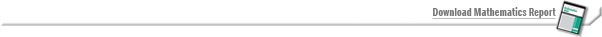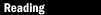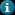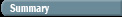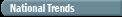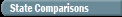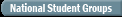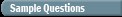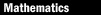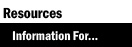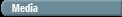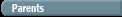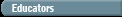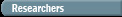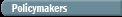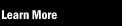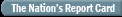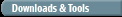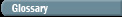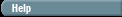Test Yourself Sample Questions: Mathematics

The following questions were included in the 2005 NAEP mathematics assessment at grade 8. As in the actual assessment, some of the questions are multiple-choice, and others are open-ended constructed-response items. Although students are asked to hand-write their responses, you can type yours in the space provided.

Question 1: In the equation y = 4x if the value of x is increased by 2, what is the effect on the value of y ?

 A. It is 8 more than the original amount. B. It is 6 more than the original amount. C. It is 2 more than the original amount. D. It is 16 times the original amount. E. It is 8 times the original amount.

Question 2: There were 90 employees in a company last year. This year the number of employees increased by 10 percent. How many employees are in the company this year?

 A. 9 B. 81 C. 91 D. 99 E. 100

Question 3: How many of the angles in this triangle are smaller than a right angle?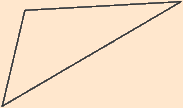A. None B. One C. Two D. Three

Question 4: The prices of gasoline in a certain region are \$1.41, \$1.36, \$1.57, and \$1.45 per gallon. What is the median price per gallon for gasoline in this region?

 A. \$1.41 B. \$1.43 C. \$1.44 D. \$1.45

Question 5: Raynold had 31 baseball cards. He gave the cards to his friends. Six of his friends received 3 cards each. Seven of his friends received 1 card each. The rest received 2 cards each. How many of his friends received exactly 2 cards from Raynold?